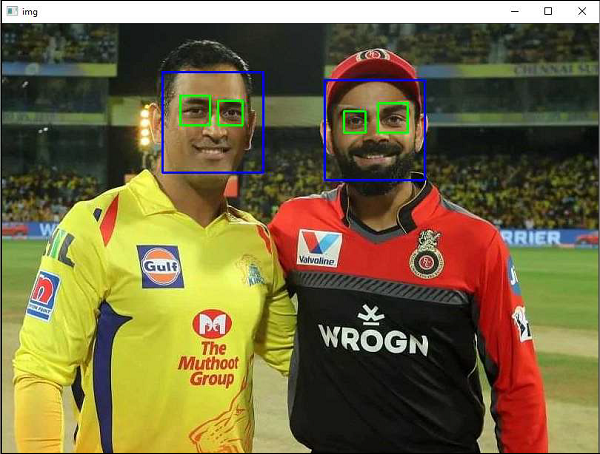# OpenCV Python - Face Detection

OpenCV uses Haar feature-based cascade classifiers for the object detection. It is a machine learning based algorithm, where a cascade function is trained from a lot of positive and negative images. It is then used to detect objects in other images. The algorithm uses the concept of Cascade of Classifiers.

The DetectMultiScale() method of CascadeClassifier class detects objects in the input image. It returns the positions of detected faces as in the form of Rectangle and its dimensions (x,y,w,h). Once we get these locations, we can use it for eye detection since eyes are always on the face!

## Example

The complete code for face detection is as follows −

import numpy as np
import cv2

gray = cv2.cvtColor(img, cv2.COLOR_BGR2GRAY)
for (x,y,w,h) in faces:
img = cv2.rectangle(img,(x,y),(x+w,y+h),(255,0,0),2)
roi_gray = gray[y:y+h, x:x+w]
roi_color = img[y:y+h, x:x+w]
for (ex,ey,ew,eh) in eyes:
cv2.rectangle(roi_color,(ex,ey),(ex+ew,ey+eh),(0,255,0),2)

cv2.imshow('img',img)
cv2.waitKey(0)
cv2.destroyAllWindows()


## Output

You will get rectangles drawn around faces in the input image as shown below −